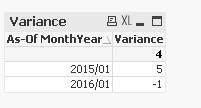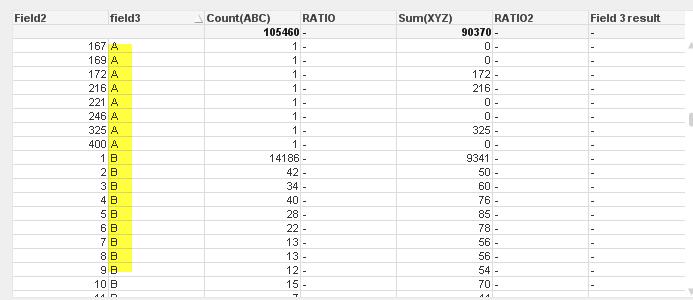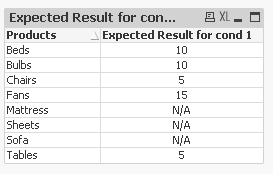# New to QlikView

Discussion board where members can get started with QlikView.

Contributor II

## Help with writing expression

Hello everyone,

I need help with writing the following conditions in QV expression of straight table.

Ratio1=  If ([ABC]=0) Then 0 Else ([feild2]/[ABC])

ABC= count(field1)

Ratio2= If([ABC]=0) Then 0 Else ([XYZ]/[ABC])

ABC= count(field1)

XYZ= sum(field2)

UVW =If(([Count(field)]-[field2])=0) Then 0 Else ([field3]/([Count(field1)]-[field2]))

LMN = If( ([field 1] In List ("A" ; "B")) )Then "10" Else If( ([field1] InList("D" ; "F")) )Then "15"  ElseIf( ([field1]="C") )Then "12"  Else "N/A"

1 Solution

Accepted SolutionsMVP

## Re: Help with writing expression

4th and final requirementPay close attention to the data model here. As I added an As-of Table (The As-Of Table)

16 RepliesMVP

## Re: Help with writing expression

Would you be able to share some data with expected output? It would be easier to resolve this with the data, rather then trying to decode your provided codeContributor II

## Re: Help with writing expression

Hi Sunny,

I tried to provide some data through screenshots. Hope it worksIn this ,   Count (ABC) and field 2 has its values in #’s.

In the Ratio column, I would expect to see that: if Count(ABC) is ‘0’ then  RATIO should display ‘0’ orelse it should display (33/3) which is 11. I want to see '11' in RATIO column

Ratio2 I would like to see ‘0’ if Count(ABC)=0 orelse  it should display Sum(XYZ)/Count(ABC)

66/3= 22'Field 3 result' what I want to see is If field 3 is A or B then result should show ‘10’, if field 3 is ‘C’ result should show 12, if field 3 is D or F, result should show 15 if field 3 is not A,B,C,D,F result should show’N/A’

ThanksMVP

## Re: Help with writing expression

Can you try this for RATIO -> Alt((Field2/Count(ABC)), 0)

and RATIO2 -> Alt((Sum(XYZ)/Count(ABC)), 0)

Contributor II

## Re: Help with writing expression

Hi Sunny,

I am not able to see the expected results using the above expressions.

I will try to create some test data and share it with you so that you will be able to help me.

ThanksMVP

## Re: Help with writing expression

Ya that would be very helpfulContributor II

## Re: Help with writing expression

Hi Sunny, I have attached the files with some sample data.

Condition 1:  expected result

If Rating is either A or B result should show ‘5’

If Rating is either D or F result should show ‘10’

If Rating is C result should show ‘15’

If rating is neither A nor B nor D nor F nor C then result should show ‘N/A’

Condition2 : expected result

If Rating is null, it should show ‘ Not Rated’

If Rating is either D or F it should show  that particular rating –ABC Avg

If 😧 D-ABC Avg

If F: F-ABC Avg

Anything apart from above conditions it should show that particular rating  Avg

Example: if rating is G it should show : G Avg

Condition 3 expected result( %): Event Example.qvw

What I am expecting in the result is :

We have count (ID) of Event A =2 and Total  count of ID’s= 5

Expected result = 2/5*100= 40%

Similarly Event B expected result= 3/5*100= 60%

ThanksMVP

## Re: Help with writing expression

For condition 1, you can use this expression:

=If(IsNull(RATING), 'N/A', Pick(Match(RATING, 'A', 'B', 'C', 'D', 'F') + 1, 'N/A', 5, 5, 15, 10, 10))For condition 2, where do I get the numbers from? Condition1?

Contributor II

## Re: Help with writing expression

Hi Sunny, Condition 1 works. Yes, same data for condition2.

Thanks

Contributor II

## Re: Help with writing expression

Hi Sunny, any update on the 2nd and 3rd condition?

I tried this for condition 2:

=If(IsNull(Rating),'Not Rated', if(Rating= Valuelist( ‘D’,'F'),Concat(Rating,'-PAR Avg'),Concat(Rating,'Avg')))

But it is not working. Please suggest

Also, I need help with one more condition.

Condition4:

If I select 2016/01, the variance should show Count(2016/01 Request ID) - Count(2015/01 Request ID)

Example: 2016/01 Count( Request ID= 4 and Count(2015/01 Request ID ) = 5

Variance= 4-5= -1

What I am trying to achieve is I am calculating the variance of current year and prior year for the same month. Attached is some sample info.